# Bayesian Logistic Regression

I recently had to run an experiment with Bayesian Logistic Regression but didn’t find a place that contained both a satisfying explanation for the motivation and derivation of the gradient.

We assume that the class label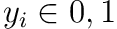can be predicted from the input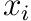and some shared latent variable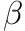through the logistic function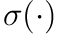,This allows us to predict new points by marginalizing out the inferred distribution over,We don’t know the posterior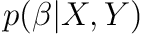though. How can we learn this distribution? By Bayes rule we obtain,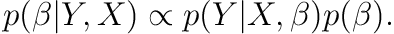##### MLE

A common way to estimate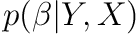is by a point estimate through maximum likelihood estimation (MLE). This is simply using the mode of the log-likelihood (effectively assuming uniform prior on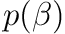),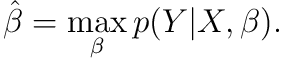For logistic regression there is no close form solution for the above but the negative log-likelihood is convex and positive definite so standard optimization techniques applies to find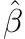. But what if we wanted to get the distribution over?

##### Approximation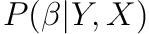has no nice parametric form that we know. Usually two approaches are considered:

• Decide on a deterministic method to obtain an approximation. If this lives within some chosen parametric family it is called variational inference. the Laplace approximation which construct a Gaussian around the mode ofcan also be considered such a method.
• Use MCMC to draw samples fromto construct a linear combination of point estimates as the approximation.

For either approaches the gradient is usually required.

## 2 Derivatives of log-likelihood

In all the approaches we needed to work with the log-likelihood. Specifically, we require the gradient. Assuming i.i.d. samples we can write the likelihood as,This correctly “activates” either of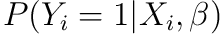and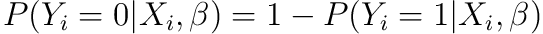depending on the value of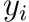.

The log-likelihood is trivially,The derivative of the log-likelihood (sometimes called the score) can then be shown to be,## 3 In practice

The way we have defined the log-likelihood we need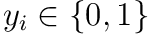for things to work out nicely.

## 4 Connection with optimization

There is a nice connection with regularization if we instead of MLE did maximum a posteriori (MAP) which simply maximizes,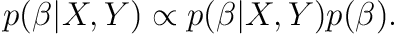Working with the potential functions instead (i.e. the log probability),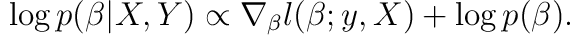If we choose a Gaussian prior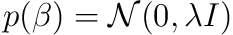this is,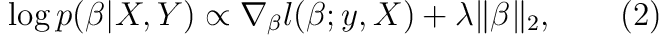Which is simply the negative log-likelihood with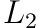-regularization!

## 5 Alternatives

The two assumptions we made in the beginning can be altered:

• The logistic function can we exchange with another sigmoid function, e.g. the standard normal cumulative distribution.
• We could choose a different prior than Gaussian. However, it is particularly nice computationally since it makes the optimization objective strongly convex. Even if we try to sample the posterior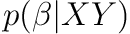instead of optimizing with MAP this is desirable. This is because gradient based sampling techniques such as Unadjusted Langevin Dynamics (ULA) similarly benefits from the strong convexity (see e.g. ).

## 6 Resources

A resource I found useful was Roman Garnett lecture notes.

## 7 Exercises

1. Derive the gradient of the log-likelihood in eq. 1.
2. Verify that Gaussian is equivalent to-regularization in eq. 2.

 A. Durmus and S. Majewski, “Analysis of langevin monte carlo via convex optimization.” Journal of Machine Learning Research, vol. 20, no. 73, pp. 1–46, 2019.